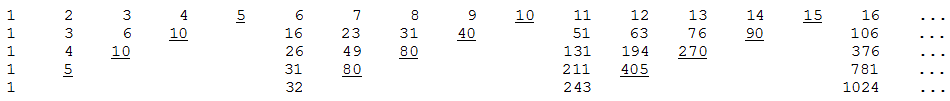# Moessner’s TheoremWrite out the positive integers in a row and underline every fifth number. Now ignore the underlined numbers and record the partial sums of the other numbers in a second row, placing each sum directly beneath the last entry that it contains.

Now, in this second row, underline and ignore every fourth number, and record the partial sums in a third row. Keep this up and the entries in the fifth row will turn out to be the perfect fifth powers 15, 25, 35, 45, 55

If we’d started by ignoring every fourth number in the original row, we’d have ended up with perfect fourth powers. In fact,

For every positive integer k > 1, if every kth number is ignored in row 1, every (k – 1)th number in row 2, and, in general, every (k + 1 – i)th number in row i, then the kth row of partial sums will turn out to be just the perfect kth powers 1k, 2k, 3k

This was discovered in 1951 by Alfred Moessner, a giant of recreational mathematics who published many such curiosa in Scripta Mathematica between 1932 and 1957.

(Ross Honsberger, More Mathematical Morsels, 1991.)